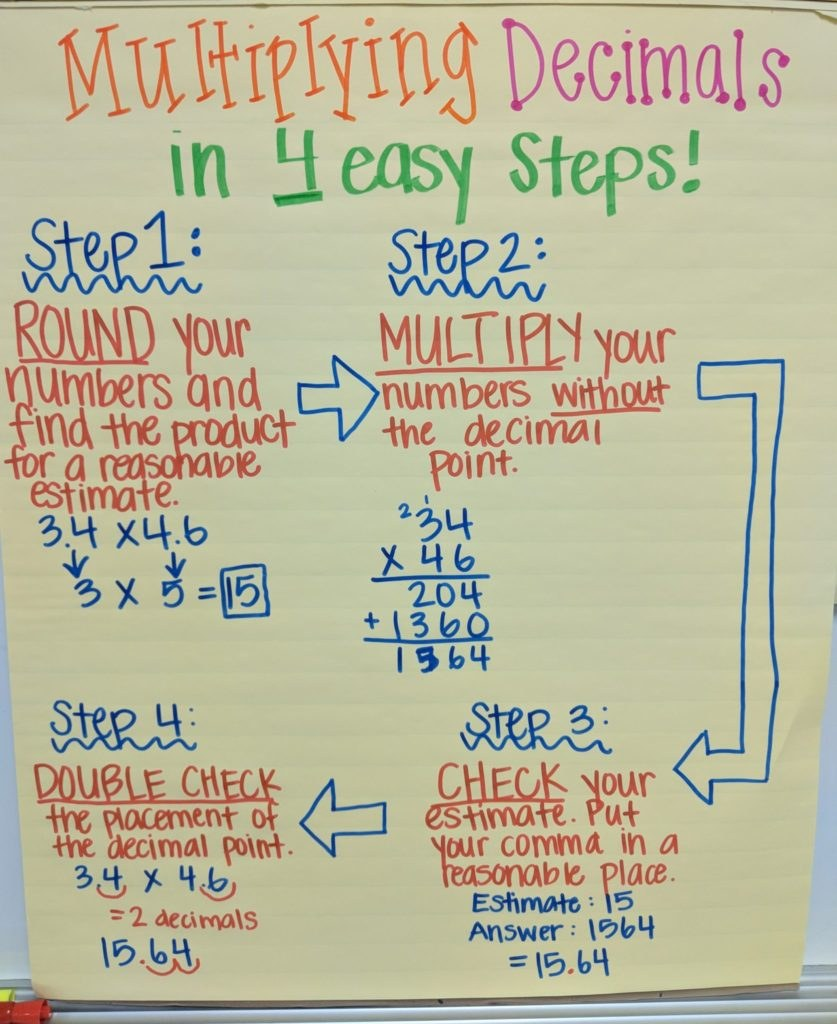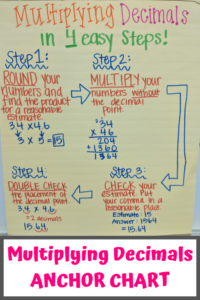# Multiplying Decimals Anchor Chart for Elementary Math Classroom

Teaching elementary math students to multiply decimals can be difficult for us teachers!  So often, students see a decimal point and they forget that multiplying decimals isn’t a foreign concept if they just take a moment to think about the process.  And there is no reason to just place the decimal randomly!

To help my students remember how to multiply decimals, I made this multiplying decimals anchor chart.  It hangs on the wall of my classroom.  When students now see decimals, I’ll find them looking in the direction of the anchor chart and organizing their thoughts rather than freaking out.

I find it helpful to create the anchor chart along with the students so they can be a part of the process.  If it’s a little messy during class creation, you can always make a separate one after school’s out.   I then hang the anchor chart on the wall for students to refer back to as we are learning and practicing the concept.

## Here’s My Multiplying Decimals Anchor ChartStudents need to understand the concept of decimal multiplication along with the rules.  When tackling a seemingly complex problem such as decimal multiplication, I find it easier to break the process down into small, manageable steps that will help them understand.  That’s why this multiplying decimals anchor chart has 4 simple steps.

## Multiplying Decimals In 4 Easy Steps;

Here are the steps that I encourage students to follow when multiplying.  As an example in my anchor chart, I show how to multiply 3.4×4.6.

1. First, round each decimal to the nearest whole number.  Then, multiply those two numbers to get a reasonable estimate.  The example in my multiplying decimals anchor chart is 3.4 rounds to 3, and 4.6 rounds to 5.  Thus 3×5=15, which is a good starting estimate for the answer.
2. After getting the estimate, multiply the non-rounded numbers without the decimal point.  Thus, multiply 34×46.  This gives a product of 1564.
3. At this point, refer back to your original estimate.  Put your decimal in a reasonable place.  Meaning, if you came up with 1564, it’s only reasonable your decimal goes between the 5 and the 6.  Because 15.64 would be the closest answer to 15.  (I don’t know why I wrote comma in my anchor chart on this step! Mom brain!)
4. Double-check the placement of your decimal point.  Do this by figuring out how many places after the decimal point of each starting number first, and add those together.  Each of my staring numbers has 1 number after the decimal point, so this is a total of 2 places.  Thus, the final multiplication product needs to have 2 numbers after the decimals.

## How This Multiplying Decimals Anchor Chart Has Worked In My Classroom

This decimal multiplication anchor chart has helped my students a lot with this concept. Instead of jumping ahead to multiplying the whole numbers and then randomly placing the decimal, my students now slow down a little more to try and understand the concept behind decimal multiplication.  I still find some students willy-nilly throwing around decimal points, but overall, I’ve seen a definite improvement!

This anchor chart is one of my favorite math anchor charts that I use in my math classroom if you’re wanting to check out other anchor charts I use in my third and fifth-grade math classrooms.